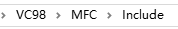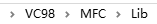7 篇文章 7 订阅

→【C语言实现动画控制】←

# 1.素材准备

1. easyx的下载链接如下：（本文使用的版本是2014冬至版）
注：使用easyx需要注意它兼容的编译器（下载的帮助文件会写），不同的easyx兼容的编译器不同，但总是和visual C++6兼容（和字符编码有关），本文以visual C++6编译器为例书写代码。
2. easyx的最新英文帮助文档链接（下载2014冬至版会自带中文帮助文档）：
https://docs.easyx.cn/en-us/intro
3. 如果你成功下载了easyx2014冬至版，那么解压后把头文件（easyx.h和graphic.h）和lib文件（amd64）分别放在VC文件夹默认的include文件夹和lib文件夹中。右键你的VC程序，选择打开文件所在位置，然后找到MFC文件夹，友情提供两个文件夹的位置截图。4. 建议编译的C文件以cpp后缀保存。

# 2.编程

## 2.1.创建你的界面

#include <graphics.h>              // 引用图形库头文件
#include <conio.h>
#include <stdio.h>
#include <windows.h>				//用到了定时函数sleep()
#include <math.h>

int main()
{
int i;
short win_width,win_height;//定义窗口的宽度和高度
win_width = 480;win_height = 360;
initgraph(win_width,win_height);//初始化窗口（黑屏）
for(i=0;i<256;i+=5)
{
setbkcolor(RGB(i,i,i));//设置背景色，原来默认黑色
cleardevice();//清屏（取决于背景色）
Sleep(15);//延时15ms
}
closegraph();//关闭绘图界面
}



\qquad 这段代码很容易理解，运行这段程序，就会出现逐渐明亮的屏幕。因为不断刷新背景色为 R G B ( i , i , i ) RGB(i,i,i) 。C语言中的颜色使用十六进制表示的，RGB函数可以将0~255范围内的三个整数三原色转换成这个十六进制。

## 2.2.创建按钮

#include <graphics.h>              // 引用图形库头文件
#include <conio.h>
#include <stdio.h>
#include <windows.h>				//用到了定时函数sleep()
#include <math.h>
int r1[]={30,20,130,60};//输入按钮的矩形参数
int r2[]={170,20,220,60};//运行按钮的矩形参数
int r3[]={260,20,310,60};//退出按钮的矩形参数
int main()
{
int i;
short win_width,win_height;//定义窗口的宽度和高度
win_width = 480;win_height = 360;
initgraph(win_width,win_height);//初始化窗口（黑屏）
for(i=0;i<256;i+=5)
{
setbkcolor(RGB(i,i,i));//设置背景色，原来默认黑色
cleardevice();//清屏（取决于背景色）
Sleep(15);//延时15ms
}
RECT R1={r1,r1,r1,r1};//矩形指针R1
RECT R2={r2,r2,r2,r2};//矩形指针R2
RECT R3={r3,r3,r3,r3};//矩形指针R3
LOGFONT f;//字体样式指针
gettextstyle(&f);					//获取字体样式
_tcscpy(f.lfFaceName,_T("宋体"));	//设置字体为宋体
f.lfQuality = ANTIALIASED_QUALITY;    // 设置输出效果为抗锯齿
settextstyle(&f);                     // 设置字体样式
settextcolor(BLACK);				//BLACK在graphic.h头文件里面被定义为黑色的颜色常量
drawtext("输入参数",&R1,DT_CENTER | DT_VCENTER | DT_SINGLELINE);//在矩形区域R1内输入文字，水平居中，垂直居中，单行显示
drawtext("运行",&R2,DT_CENTER | DT_VCENTER | DT_SINGLELINE);//在矩形区域R2内输入文字，水平居中，垂直居中，单行显示
drawtext("退出",&R3,DT_CENTER | DT_VCENTER | DT_SINGLELINE);//在矩形区域R3内输入文字，水平居中，垂直居中，单行显示
setlinecolor(BLACK);
rectangle(r1,r1,r1,r1);
rectangle(r2,r2,r2,r2);
rectangle(r3,r3,r3,r3);
system("pause");//暂停，为了显示
closegraph();
return 0;
}\qquad 这里需要特别介绍的是矩形指针 p R e c t pRect ，它使用句柄RECT定义，并且不可以中途再次赋值。之所以要设置矩形指针了为了打印字体的时候以矩形为边界自动填充。它的格式是RECT r={X1,Y1,X2,Y2}，X1和X2是矩形的左边和右边的横坐标，Y1和Y2是矩形的上边和下边的纵坐标，这一点和rectangle()绘制空心矩形函数参数排列一致。后面的DT_CENTER | DT_VCENTER | DT_SINGLELINE就是描述填充格式的常量。使用drawtext书写文字不需要再计算文字的坐标和设置大小，会方便很多。

## 2.3.鼠标操作

### 2.3.1.单击特效

• WM_MOUSEMOVE——鼠标移动
• WM_MOUSEWHEEL——鼠标滚轮滚动
• WM_LBUTTONDOWN——鼠标左键按下
• WM_LBUTTONUP——鼠标左键弹起
• WM_LBUTTONDBLCLK——鼠标左键双击
• WM_RBUTTONDOWN——鼠标右键按下
• WM_RBUTTONUP——鼠标右键弹起
• WM_RBUTTONDBLCLK——鼠标左键双击
• WM_MBUTTONDOWN——鼠标中键按下
• WM_MBUTTONUP——鼠标中键弹起
• WM_MBUTTONDBLCLK——鼠标中键双击

#include <graphics.h>              // 引用图形库头文件
#include <conio.h>
#include <stdio.h>
#include <windows.h>				//用到了定时函数sleep()
#include <math.h>
int r1[]={30,20,130,60};//输入按钮的矩形参数
int r2[]={170,20,220,60};//运行按钮的矩形参数
int r3[]={260,20,310,60};//退出按钮的矩形参数
int main()
{
int i;
short win_width,win_height;//定义窗口的宽度和高度
win_width = 480;win_height = 360;
initgraph(win_width,win_height);//初始化窗口（黑屏）
for(i=0;i<256;i+=5)
{
setbkcolor(RGB(i,i,i));//设置背景色，原来默认黑色
cleardevice();//清屏（取决于背景色）
Sleep(15);//延时15ms
}
RECT R1={r1,r1,r1,r1};//按钮1的矩形区域
RECT R2={r2,r2,r2,r2};//按钮2的矩形区域
RECT R3={r3,r3,r3,r3};//按钮2的矩形区域
LOGFONT f;
gettextstyle(&f);					//获取字体样式
_tcscpy(f.lfFaceName,_T("宋体"));	//设置字体为宋体
f.lfQuality = ANTIALIASED_QUALITY;    // 设置输出效果为抗锯齿
settextstyle(&f);                     // 设置字体样式
settextcolor(BLACK);				//BLACK在graphic.h头文件里面被定义为黑色的颜色常量
drawtext("输入参数",&R1,DT_CENTER | DT_VCENTER | DT_SINGLELINE);//在矩形区域R1内输入文字，水平居中，垂直居中，单行显示
drawtext("运行",&R2,DT_CENTER | DT_VCENTER | DT_SINGLELINE);//在矩形区域R2内输入文字，水平居中，垂直居中，单行显示
drawtext("退出",&R3,DT_CENTER | DT_VCENTER | DT_SINGLELINE);//在矩形区域R3内输入文字，水平居中，垂直居中，单行显示
setlinecolor(BLACK);
rectangle(r1,r1,r1,r1);
rectangle(r2,r2,r2,r2);
rectangle(r3,r3,r3,r3);
MOUSEMSG m;//鼠标指针
setrop2(R2_NOTXORPEN);//二元光栅——NOT(屏幕颜色 XOR 当前颜色)
while(true)
{
m = GetMouseMsg();//获取一条鼠标消息
if(m.uMsg==WM_LBUTTONDOWN)
{
for(i=0;i<=10;i++)
{
setlinecolor(RGB(25*i,25*i,25*i));//设置圆颜色
circle(m.x,m.y,2*i);
Sleep(25);//停顿2ms
circle(m.x,m.y,2*i);//抹去刚刚画的圆
}
FlushMouseMsgBuff();//清空鼠标消息缓存区
}
}
system("pause");//暂停，为了显示
closegraph();
return 0;
}\qquad 每响应一次鼠标左键单击事件，都会调用一次清空鼠标消息缓存区的函数FlushMouseMsgBuff()，如果没有这个函数会怎么样呢？如果我们快速连续地单击鼠标左键N次，那么特效就会播放N次，如果特效播放速度比单击的速度慢，那么即使你停下来了，程序仍然会接着播放单击特效，因为你的左键单击仍然在鼠标的消息队列m.uMsg中的鼠标消息没有响应完。
\qquad 这里需要解释的是一个二元光栅设置函数setrop2()，二元光栅是混合背景色和当前颜色的模式。我们这里采用的方式是同或（NOT XOR）的方式，若底色为白色（1），则当前颜色不变；若底色是黑色（0），则当前颜色反色。为什么需要采用这种方式呢？因为我们在第二次抹去原来的圆的时候不能采用白色，否则如果背景色原来就为黑（比如按钮和文字），就也会被抹成白色。而背景色与任意一个颜色同或两次都为其本身，即可起到还原背景色的效果。这里的背景色与cleardevice()前面那个背景色不同，这里的是指执行这一条绘画指令之前屏幕上的颜色。

### 2.3.2.光标感应

#include <graphics.h>              // 引用图形库头文件
#include <conio.h>
#include <stdio.h>
#include <windows.h>				//用到了定时函数sleep()
#include <math.h>
int r={{30,20,130,60},{170,20,220,60},{260,20,310,60}};//三个按钮的二维数组

int button_judge(int x,int y)
{
if(x>r && x<r && y>r && y<r)return 1;
if(x>r && x<r && y>r && y<r)return 2;
if(x>r && x<r && y>r && y<r)return 3;
return 0;
}
int main()
{
int i,event=0;
short win_width,win_height;//定义窗口的宽度和高度
win_width = 480;win_height = 360;
initgraph(win_width,win_height);//初始化窗口（黑屏）
for(i=0;i<256;i+=5)
{
setbkcolor(RGB(i,i,i));//设置背景色，原来默认黑色
cleardevice();//清屏（取决于背景色）
Sleep(15);//延时15ms
}
RECT R1={r,r,r,r};
RECT R2={r,r,r,r};
RECT R3={r,r,r,r};
LOGFONT f;
gettextstyle(&f);					//获取字体样式
_tcscpy(f.lfFaceName,_T("宋体"));	//设置字体为宋体
f.lfQuality = ANTIALIASED_QUALITY;    // 设置输出效果为抗锯齿
settextstyle(&f);                     // 设置字体样式
settextcolor(BLACK);				//BLACK在graphic.h头文件里面被定义为黑色的颜色常量
drawtext("输入参数",&R1,DT_CENTER | DT_VCENTER | DT_SINGLELINE);//在矩形区域R1内输入文字，水平居中，垂直居中，单行显示
drawtext("运行",&R2,DT_CENTER | DT_VCENTER | DT_SINGLELINE);//在矩形区域R2内输入文字，水平居中，垂直居中，单行显示
drawtext("退出",&R3,DT_CENTER | DT_VCENTER | DT_SINGLELINE);//在矩形区域R3内输入文字，水平居中，垂直居中，单行显示
setlinecolor(BLACK);
rectangle(r,r,r,r);
rectangle(r,r,r,r);
rectangle(r,r,r,r);
MOUSEMSG m;//鼠标指针

while(true)
{
m = GetMouseMsg();//获取一条鼠标消息

switch(m.uMsg)
{
case WM_MOUSEMOVE:
setrop2(R2_XORPEN);
setlinecolor(LIGHTCYAN);//线条颜色为亮青色
setlinestyle(PS_SOLID, 3);//设置画线样式为实现，10磅
setfillcolor(WHITE);//填充颜色为白色
if(button_judge(m.x,m.y)!=0)
{
if(event != button_judge(m.x,m.y))
{
event = button_judge(m.x,m.y);//记录这一次触发的按钮
fillrectangle(r[event-1],r[event-1],r[event-1],r[event-1]);//有框填充矩形（X1,Y1,X2,Y2）
}
}
else
{
if(event != 0)//上次触发的按钮未被修正为原来的颜色
{
fillrectangle(r[event-1],r[event-1],r[event-1],r[event-1]);//两次同或为原来颜色
event = 0;
}
}
break;
case WM_LBUTTONDOWN:
setrop2(R2_NOTXORPEN);//二元光栅——NOT(屏幕颜色 XOR 当前颜色)
for(i=0;i<=10;i++)
{
setlinecolor(RGB(25*i,25*i,25*i));//设置圆颜色
circle(m.x,m.y,2*i);
Sleep(30);//停顿30ms
circle(m.x,m.y,2*i);//抹去刚刚画的圆
}
break;
FlushMouseMsgBuff();//清空鼠标消息缓存区
}
}
system("pause");//暂停，为了显示
return 0;
}\qquad 这里我们运用了两次设置二元光栅的函数setrop2，在鼠标的移动条件内(case MOUSEMOVE)，我们使用的是屏幕颜色和当前颜色异或，这是为什么呢？因为fillrectangle()函数是画一个有框填充矩形，我们让这个有框填充矩形和原按钮的一样大。由于线条的颜色为亮青色，填充颜色为白色（1），白色的填充颜色和屏幕颜色异或，取的是屏幕颜色的反色。按钮的边框是黑色（0），它与亮青色异或，则会保留原来的亮青色。

### 2.3.3.进度条

\qquad 假设球半径为R，初始高度为 h 0 h_0 ，初速度为0（自由落体），非弹性碰撞时能量损失率为 α \alpha 。计算部分子函数如下：

int simulation()
{
float dt = 0.01;//仿真间隔10ms
long int N = (long int)(sim_t/dt);//迭代次数
float *h=(float*)calloc(N,sizeof(float));//高度
float *v=(float*)calloc(N,sizeof(float));//速度（竖直方向）
long int i;//迭代变量
for(i=1;i<N;i++)
{
if(h[i-1]>R)//未发生碰撞
{
v[i]=v[i-1]-9.8*dt;//速度计算
}
else//发生碰撞，动能损失alpha，速度损失alpha的开方
{
v[i]=-sqrt(alpha)*v[i-1];
}
}
free(h);
free(v);//释放内存
return 0;
}


void init_figure()
{
int i;
setrop2(R2_COPYPEN);//当前颜色
setlinecolor(BLACK);
setlinestyle(PS_SOLID);//实线
rectangle(30,100,420,330);//外框线
setlinestyle(PS_DOT);//点线
for(i=30+39;i<420;i+=39)
{
line(i,100,i,330);//竖直辅助线
}
for(i=100+23;i<330;i+=23)
{
line(30,i,420,i);//水平辅助线
}
}


int simulation()
{
float dt = 0.01;//仿真间隔10ms
float dy = 230/h0;//单位纵坐标
long int N = (long int)(sim_t/dt);//迭代次数
float *h=(float*)calloc(N,sizeof(float));//高度
float *v=(float*)calloc(N,sizeof(float));//速度（竖直方向）
long int i;//迭代变量
float process_duty;//进度
init_figure();//初始化图像网格
setrop2(R2_COPYPEN);//当前颜色
//计算步骤
h=h0;v=0;
for(i=1;i<N;i++)
{
if(h[i-1]>R)//未发生碰撞
{
v[i]=v[i-1]-9.8*dt;//速度计算
}
else//发生碰撞，动能损失alpha，速度损失alpha的开方
{
v[i]=-sqrt(alpha)*v[i-1];
}
h[i]=h[i-1]+v[i]*dt;//高度计算
process_duty = (i+1)/(float)(N);
putpixel(30+(int)(process_duty*390),330-(int)(h[i]*dy),RED);//画点putpixel(X,Y,color*)
Sleep(dt*1000);//延时
}
free(h);
free(v);
return 0;
}


\qquad 这里的新函数putpixel（X,Y,color*）是画像素点的函数，适合刻画不连续或不规则的移动轨迹。

//仿真运行
int simulation()
{
char t;//百分值的字符
char *out_text;//带百分号的百分字符
float dt = 0.01;//仿真间隔10ms
float dy = 230/h0;//单位纵坐标
long int N = (long int)(sim_t/dt);//迭代次数
float *h=(float*)calloc(N,sizeof(float));//高度
float *v=(float*)calloc(N,sizeof(float));//速度（竖直方向）
long int i;//迭代变量
float process_duty;//进度
RECT r={370,35,400,65};//百分值显示区域的矩形指针
init_figure();//初始化图像网格
setrop2(R2_COPYPEN);//当前颜色
setfillcolor(WHITE);
setlinecolor(WHITE);
fillrectangle(354,19,411,81);//覆盖原进度条区域
setlinestyle(PS_NULL);//无线条
setbkmode(TRANSPARENT);//设置文字填充背景为透明
//计算步骤
h=h0;v=0;
BeginBatchDraw();//开始缓存区
for(i=1;i<N;i++)
{
if(h[i-1]>R)//未发生碰撞
{
v[i]=v[i-1]-9.8*dt;//速度计算
}
else//发生碰撞，动能损失alpha，速度损失alpha的开方
{
v[i]=-sqrt(alpha)*v[i-1];
}
setfillcolor(WHITE);
setlinecolor(WHITE);
fillrectangle(354,19,416,81);//覆盖原进度条区域
h[i]=h[i-1]+v[i]*dt;//高度计算
process_duty = (i+1)/(float)(N);
setlinestyle(PS_SOLID);
putpixel(30+(int)(process_duty*390),330-(int)(h[i]*dy),RED);
setfillcolor(BLUE);
setlinestyle(PS_NULL);
fillpie(355,20,415,80,0,process_duty*2*PI);
setfillcolor(WHITE);
fillcircle(385,50,20);
sprintf(t,"%d",(int)(process_duty*100.0));//整型转换为字符串
out_text = strcat(t,"%");//添加一个百分号
drawtext(out_text,&r,DT_CENTER | DT_VCENTER | DT_SINGLELINE);
Sleep(dt*1000);
FlushBatchDraw();//刷新缓存区
}
EndBatchDraw();//结束缓存区
free(h);
free(v);
return 0;
}


\qquad 首先需要计算进度条的坐标，把环形进度条区域用白色矩形刷新掉，环形进度条需要一个扇形和圆形的组合，扇形的角度是0~360°。这里我们用到了fillpie(X1,Y1,X2,Y2,start_angle,end_angle)，前四个参数为椭圆扇形的外接矩形坐标，后两个参数分别为起始角和终止角（弧度制）。每过一次迭代都重新计算终止角（起始角始终为0），即可起到扇形角度逐渐增长的效果，再用一个白色填充圆覆盖中心部分即可变成环形进度条。
\qquad FlushBatchDraw()函数是刷新缓存区的函数，与BeginBatchDraw()EndBatchDraw()一起使用，如果我们绘图之后不想立即显示，而想批量绘图最后一起刷新画板，用缓存区的方法再合适不过了。

# 3.完整代码及效果

#include <graphics.h>              // 引用图形库头文件
#include <conio.h>
#include <stdio.h>
#include <windows.h>				//用到了定时函数sleep()
#include <math.h>
#include <string.h>
#define PI 3.1416
int r={{30,20,130,60},{170,20,220,60},{260,20,310,60}};//三个按钮的二维数组
float alpha,R,h0,sim_t;//碰撞时的能量损失率，球的半径、初始高度、仿真时间
//按钮判断函数
int button_judge(int x,int y)
{
if(x>r && x<r && y>r && y<r)return 1;
if(x>r && x<r && y>r && y<r)return 2;
if(x>r && x<r && y>r && y<r)return 3;
return 0;
}
//初始化图像
void init_figure()
{
int i;
setrop2(R2_COPYPEN);//当前颜色
setlinecolor(BLACK);
setlinestyle(PS_SOLID);//实线
rectangle(30,100,420,330);//外框线
setlinestyle(PS_DOT);//点线
for(i=30+39;i<420;i+=39)
{
line(i,100,i,330);//竖直辅助线
}
for(i=100+23;i<330;i+=23)
{
line(30,i,420,i);//水平辅助线
}
}
//仿真运行
int simulation()
{
char t;//百分值的字符
char *out_text;
float dt = 0.01;//仿真间隔10ms
float dy = 230/h0;//单位纵坐标
long int N = (long int)(sim_t/dt);//迭代次数
float *h=(float*)calloc(N,sizeof(float));//高度
float *v=(float*)calloc(N,sizeof(float));//速度（竖直方向）
long int i;//迭代变量
float process_duty;//进度
RECT r={370,35,400,65};//百分值显示区域的矩形指针
init_figure();//初始化图像网格
setrop2(R2_COPYPEN);//当前颜色
setfillcolor(WHITE);
setlinecolor(WHITE);
fillrectangle(354,19,411,81);//覆盖原进度条区域
setlinestyle(PS_NULL);//无线条
setbkmode(TRANSPARENT);//设置文字填充背景为透明
//计算步骤
h=h0;v=0;
BeginBatchDraw();//开始缓存区
for(i=1;i<N;i++)
{
if(h[i-1]>R)//未发生碰撞
{
v[i]=v[i-1]-9.8*dt;//速度计算
}
else//发生碰撞，动能损失alpha，速度损失alpha的开方
{
v[i]=-sqrt(alpha)*v[i-1];
}
setfillcolor(WHITE);
setlinecolor(WHITE);
fillrectangle(354,19,416,81);//覆盖原进度条区域
h[i]=h[i-1]+v[i]*dt;//高度计算
process_duty = (i+1)/(float)(N);
setlinestyle(PS_SOLID);
putpixel(30+(int)(process_duty*390),330-(int)(h[i]*dy),RED);
setfillcolor(BLUE);
setlinestyle(PS_NULL);
fillpie(355,20,415,80,0,process_duty*2*PI);
setfillcolor(WHITE);
fillcircle(385,50,20);
sprintf(t,"%d",(int)(process_duty*100.0));//整型转换为字符串
out_text = strcat(t,"%");//添加一个百分号
drawtext(out_text,&r,DT_CENTER | DT_VCENTER | DT_SINGLELINE);
Sleep(dt*1000);
FlushBatchDraw();//刷新缓存区
}
EndBatchDraw();//结束缓存区
free(h);
free(v);
return 0;
}

int main()
{
int i,event=0;
char s;//输入字符串变量
short win_width,win_height;//定义窗口的宽度和高度
win_width = 480;win_height = 360;
initgraph(win_width,win_height);//初始化窗口（黑屏）
for(i=0;i<256;i+=5)
{
setbkcolor(RGB(i,i,i));//设置背景色，原来默认黑色
cleardevice();//清屏（取决于背景色）
Sleep(30);//延时30ms
}
RECT R1={r,r,r,r};
RECT R2={r,r,r,r};
RECT R3={r,r,r,r};
LOGFONT f;//字体样式指针
gettextstyle(&f);					//获取字体样式
_tcscpy(f.lfFaceName,_T("宋体"));	//设置字体为宋体
f.lfQuality = ANTIALIASED_QUALITY;    // 设置输出效果为抗锯齿
settextstyle(&f);                     // 设置字体样式
settextcolor(BLACK);				//BLACK在graphic.h头文件里面被定义为黑色的颜色常量
drawtext("输入参数",&R1,DT_CENTER | DT_VCENTER | DT_SINGLELINE);//在矩形区域R1内输入文字，水平居中，垂直居中，单行显示
drawtext("运行",&R2,DT_CENTER | DT_VCENTER | DT_SINGLELINE);//在矩形区域R2内输入文字，水平居中，垂直居中，单行显示
drawtext("退出",&R3,DT_CENTER | DT_VCENTER | DT_SINGLELINE);//在矩形区域R3内输入文字，水平居中，垂直居中，单行显示
setlinecolor(BLACK);
rectangle(r,r,r,r);
rectangle(r,r,r,r);
rectangle(r,r,r,r);
MOUSEMSG m;//鼠标指针

while(true)
{
m = GetMouseMsg();//获取一条鼠标消息

switch(m.uMsg)
{
case WM_MOUSEMOVE:
setrop2(R2_XORPEN);
setlinecolor(LIGHTCYAN);//线条颜色为亮青色
setlinestyle(PS_SOLID, 3);//设置画线样式为实现，10磅
setfillcolor(WHITE);//填充颜色为白色
if(button_judge(m.x,m.y)!=0)
{
if(event != button_judge(m.x,m.y))
{
event = button_judge(m.x,m.y);//记录这一次触发的按钮
fillrectangle(r[event-1],r[event-1],r[event-1],r[event-1]);//有框填充矩形（X1,Y1,X2,Y2）
}
}
else
{
if(event!=0)//上次触发的按钮未被修正为原来的颜色
{
fillrectangle(r[event-1],r[event-1],r[event-1],r[event-1]);//两次同或为原来颜色
event = 0;
}
}
break;
case WM_LBUTTONDOWN:
setrop2(R2_NOTXORPEN);//二元光栅——NOT(屏幕颜色 XOR 当前颜色)
for(i=0;i<=10;i++)
{
setlinecolor(RGB(25*i,25*i,25*i));//设置圆颜色
circle(m.x,m.y,2*i);
Sleep(20);//停顿30ms
circle(m.x,m.y,2*i);//抹去刚刚画的圆
}
//按照按钮判断左键单击后的操作
switch(button_judge(m.x,m.y))
{
//复原按钮原型
case 1:
InputBox(s,30,"请输入碰撞时的能量损失率、球的半径、初始高度、仿真时间");
sscanf(s,"%f%f%f%f",&alpha,&R,&h0,&sim_t);//将输入字符串依次扫描到全局变量里面
FlushMouseMsgBuffer();//单击事件后清空鼠标消息
break;
case 2:
simulation();//仿真运行
FlushMouseMsgBuffer();//单击事件后清空鼠标消息
break;
case 3:
closegraph();//关闭绘图环境
exit(0);//正常退出
default:
FlushMouseMsgBuffer();//单击事件后清空鼠标消息
//printf("\r\n(%d,%d)",m.x,m.y);//打印鼠标坐标，方便调试时确定区域
break;
}
break;
}
}
return 0;
}12-2807-30
03-264万+
02-012万+
11-081万+
05-17606
01-143万+
03-133万+
01-26795
12-25
12-191万+
06-261626
07-21176
05-282万+
04-245968
05-25111
01-18点击重新获取扫码支付余额充值